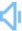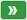Directly to word page Vague search(google)

## Divided in a sentence

Sentence count:241+42 Only show simple sentencesPosted:2017-03-26Updated:2020-07-24
Similar words: Meaning: [-dɪd]adj. 1. separated into parts or pieces 2. having a median strip or island between lanes of traffic moving in opposite directions 3. distributed in portions (often equal) on the basis of a plan or purpose.Random good picture Not show
(1) A house divided against itself cannot stand.(2) If a house be divided against itself, that house cannot stand.(3) The chinese word for crisis is divided into two characters, one meaning danger and the other meaning opportunity.(4) The party was divided on this issue.
(5) This lesson is divided into four units.
(6) Opinions are divided on this point.
(7) Manhattan is divided into distinct neighborhoods.
(8) The bag is divided into separate compartments .
(9) Opinion is deeply divided on this issue.
(10) Canada is divided into 10 provinces and 2 territories.
(11) Berlin was divided into four sectors after the war.
(12) The jury were divided in opinion.
(13) They divided the money between themselves.
(14) Nine divided by three is three.
(15) The room is divided by movable screens.
(16) Administratively,the country is divided into 50 states.
(17) I've divided the money in half.
(18) Europe is divided into economic zones.
(19) Lines divided the area into segments.
(20) The academic year is divided into two terms.
(21) The food was divided out among all the people.
(22) The cafe was divided up by glass partitions.
(23) The fields were divided by stone walls.
(24) They divided the prize fifty-fifty.
(25) A sentence can be divided up into meaningful segments. Sentencedict.com
(26) The period can be divided into three distinct phases.
(27) This play is divided into three acts, and each act has three scenes.
(28) There were four of us, so we divided the orange into quarters and each ate a piece.
(29) The teacher divided the class and took one section to the library and left the other to write an article.
(30) A bank's local market share tends to be divided equally between the local branch and branches located elsewhere.
Total 241, 30 Per page  1/9  «first  next  last»  goto